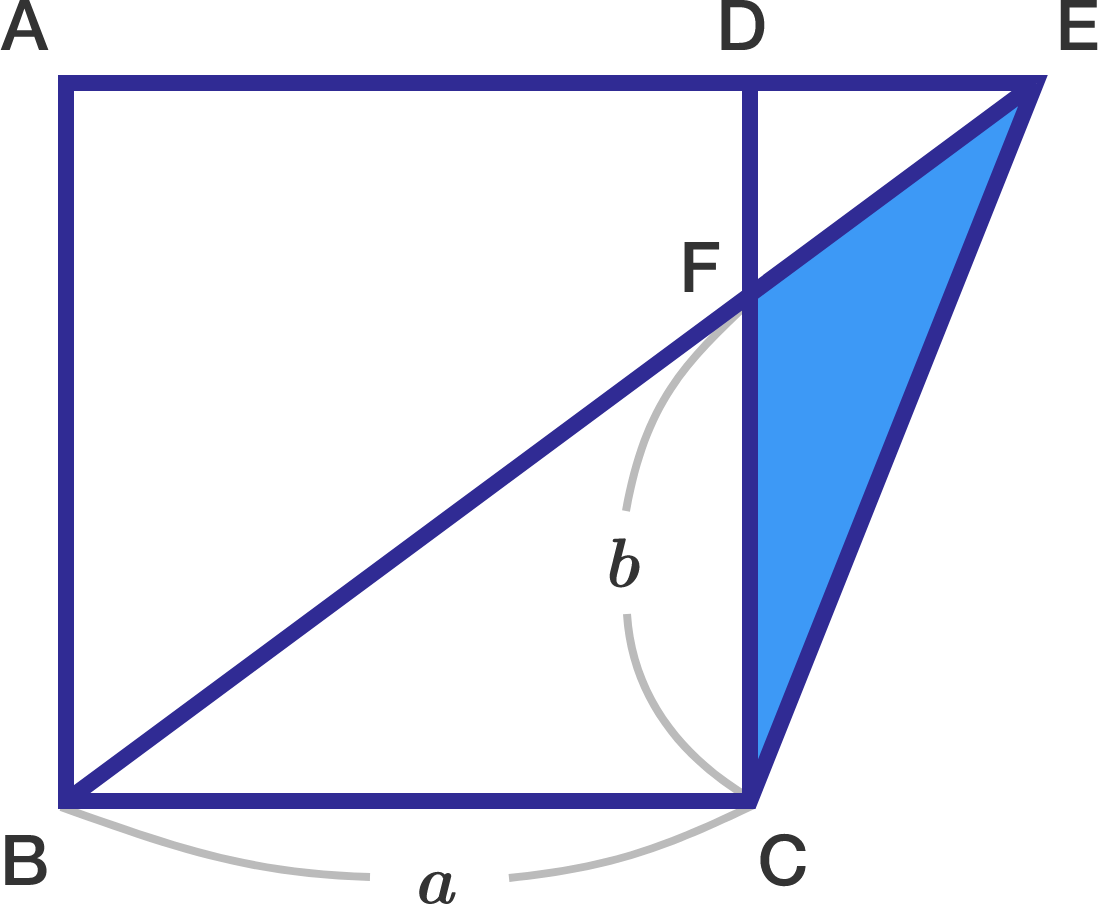# Similar Triangles Might Help?In the above diagram, $\square ABCD$ is a square with side $\overline{AD}$ extended to point $E$, and $\overline{BE}$ is a straight line. If the length of $\overline{BC}$ is $\lvert \overline{BC}\rvert = a=30$ and $\lvert \overline{CF}\rvert =b= 20,$ what is the area of $\triangle CEF?$

×